# RD Sharma Solutions Class 9 Chapter 8 Lines and Angles

Read RD Sharma Solutions Class 9 Chapter 8 Lines and Angles below, students should study RD Sharma class 9 Mathematics available on Studiestoday.com with solved questions and answers. These chapter wise answers for class 9 Mathematics have been prepared by teacher of Grade 9. These RD Sharma class 9 Solutions have been designed as per the latest NCERT syllabus for class 9 and if practiced thoroughly can help you to score good marks in standard 9 Mathematics class tests and examinations

Exercise 8.1

Question 1: Write the complement of each of the following angles:
(i) 20°
(ii) 35°
(iii) 90°
(iv) 77°
(v) 30°
Solution:
(i) Sum of complement angles = 90°
First angle = 20°
The other angle is = 90° – 20° = 70°
Hence, the other complement angle is 70°.

(ii) Sum of complement angles = 90°
First angle = 35°
The other angle is = 90° – 35° = 55°
Hence, the other complement angle is 55°.

(iii) Sum of complement angles = 90°
First angle = 90°
The other angle is = 90° – 90° = 0°
Hence, the other complement angle is 0°.

(iv) Sum of complement angles = 90°
First angle = 77°
The other angle is = 90° – 77° = 13°
Hence, the other complement angle is 13°.

(v) Sum of complement angles = 90°
First angle = 30°
The other angle is = 90° – 30° = 60°
Hence, the other complement angle is 60°.

Question 2 : Write the supplement of each of the following angles:
(i) 54°
(ii) 132°
(iii) 138°
Solution:
(i) Sum of supplement angles = 180°.
First angle = 54°
The other angle is = 180° – 54° = 126°
Hence, the other supplementary angle is 126°.

(ii) Sum of supplement angles = 180°.
First angle = 132°
The other angle is = 180° – 132° = 48°
Hence, the other supplementary angle is 48°.

(iii) Sum of supplement angles = 180°.
First angle = 138°
The other angle is = 180° – 138° = 42°
Hence, the other supplementary angle is 42°.

Question 3: If an angle is 28° less than its complement, find its measure?
Solution:
Let any angle of complementary angles be x°.
The other complementary angle will be (x+28)°
The sum of complementary angle will be 90°
90° = x+(x+28)°
90° = x+x+28°
90°-28° = x+x
62° = 2x
(62°)/2 = x
31° = x
Thus, the angle measured is 31°.

Question 4: If an angle is 30° more than one half of its complement, find the measure of the angle?
Solution:
Let any angle of complementary angles be x°.
The other complementary angle will be x/2+30°
The sum of complementary angle will be 90°
90° = x+(x/2+30)°
90° = x+x/2+30°
90°-30° = x+x/2
60° = (2x+x)/2
60×2=3x
(120°)/3=x
40°=x
Other angle = 40/2+30
Other angle = 40/2+30
Other angle = 20+30
Other angle = 50°
Thus, the measure of the angle is 50°.

Question 5: Two supplementary angles are in the ratio 4:5. Find the angles?
Solution:
The sum of supplementary angles will be180°.
It is given that the two supplementary angles are in the ratio 4:5.
Sum of the Ratios = 4 + 5 = 9
First angle = 4/9×180=80°
Second angle = 5/9×180=100°
Thus, the required angles are 80° and 100°.

Question 6: Two supplementary angles differ by 48°. Find the angles?
Solution:
Let the first angle of supplementary angle be x°.
Second angle of supplementary angle be (180 - x°).
It is given that the difference of two supplementary angles is 48°.
x-(180-x)=48
x-180+x=48
2x=48-180
2x=228
x=114
First angle will be 114°
Second angle will be 180°-114°
Second angle will be 66°
Thus, the two supplementary angles are 66° and 114°.

Question 7: An angle is equal to 8 times its complement. Determine its measure?
Solution:
Let the first angle of complementary angle be x°.
Second angle of complementary angle be (90 - x°).
It is given that an angle is equal to 8 times.
x°=8(90-x°)
x°=720-8x°
x°+ 8x° = 720
9x° = 720
x° = (720 )/9
x° = 80°
Hence, the required angle is 80°

Question 8:  If the angle (2x-10)° and (x-5)° are complementary angles. Find the value of x°.
Solution:
It is given that,
The first angle of complementary angles is (2x-10)°
The second angle of complementary angles is (x-5)°
(2x-10)°+(x-5)°=90
2x-10+x-5°=90
3x-15=90
3x=90+15
3x=105
x=105/3
x=35
Hence, the value of x is 35°.

Question 9: If the complement of an angle is equal to supplement of thrice of it find the measure of it.
Solution:
The sum of complementary angles 90°
And the sum of Supplementary angles 180°
Let the first angle of complementary angle will be x°
And the other angle be (90-x°)
Let the first angle of supplementary angle will be 3x°
And the other angle be (180-3x°)
It is given that the complement of an angle is equal to supplement of thrice
(90-x°)=(180-3x°)
90-x°=180-3x°
-x°+3x°=180-90
2x°=90°
x=(90°)/2
x=45°

Question 10: If an angle differ by is complementary by 10°. Find the angle.
Solution:
Let the first angle of complementary angle is x°
and the second angle of complementary angle is 90-x°
It is given that the difference of complementary angles is 10.
x-(90-x)=10
x-90+x=10
x+x=10+90
2x=100
x=100/2
x=50

Question 11:  If the supplement of an angle is three times its complement. Find the angle.
Solution:
The sum of complementary angles 90°
And the sum of Supplementary angles 180°
Let the first angle of complementary angle is x°
and the second angle of complementary angle is 90-x°
and the second angle of supplementary angle is 180-x°
180-x=3(90-x)
180-x=270-3x
180-270=x-3x
-90=-2x
90/2=x
45°=x

Question 12:  If the supplement of an angle is two third of its Determine the angle and its supplement.
Solution:
The sum of Supplementary angles will be 180°
Let the first angle of supplementary angle be x°.
Second angle of supplementary angle be (180 - x°)
(180-x°)=2/3 x°
3(180-x°)=2x°
540-3x°=2x°
540=2x°+3x°
540=5x°
540/5=x°
108°=x°
The other supplements are 180 –108=72°

Question 13: An angle is 14° more than its complementary angle. What is its measure?
Solution:
Let the first angle of complementary angle is x°
and the second angle of complementary angle is 90-x°
The sum of complementary angles is 90 °
x°-14=90-x°
x°+x°=90+14
2x°=90+14
2x°=104
x°=(104 )/2
x°=52°
The other supplementary angle is 52°-14=38°

Question 14: The measure of an angle is twice of its supplement angle. Find its measure.
Solution:
The sum of Supplementary angles will be 180°
Let the first angle of supplementary angle be x°.
Second angle of supplementary angle be (180-x°)
x°=2(180-x°)
x°=360-2x°
x°+2x°=360
3x°=360
x°=360/3
x°=120°

Exercise 8.2

Question 1:  In the below Fig. OA and OB are opposite rays:
(i) If x = 25°, what is the value of y?
(ii) If y = 35°, what is the value of x?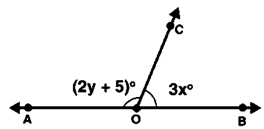Solution:
(i) It is given that the value of x = 25
∠AOC = 2y + 5 and ∠BOC = 3x
We, know that,
∠AOC + ∠BOC = 180° (Liner Pair)
(2y + 5) + 3x = 180°
Put the value of x in above equation.
(2y + 5) + 3(25) = 180°
2y + 5 + 75 = 180°
2y + 80 = 180°
2y = 100°
y = 100/2
y = 50°
Hence, the value of y = 50°

(ii) It is given that the value of y = 35°
We, know that,
∠AOC + ∠BOC = 180°
(2y + 5) + 3x = 180
Put the value of y in above equation.
(2(35) + 5) + 3x = 180
75 + 3x = 180
3x = 105
x = 35
Hence, the value of x = 35°

Question 2:  In the below figure, write all pairs of adjacent angles and all the linear pairs.Solution:
∠AOC, ∠COB;
∠AOD, ∠BOD;
∠AOD, ∠COD;
∠BOC, ∠COD
Linear pair:-
∠AOD + ∠BOD = 180°
∠AOC+ ∠BOC = 180°

Question 3: In the given figure, find x. Further find ∠BOC, ∠COD and ∠AOD.Solution:
∠AOD and ∠BOD form a linear pair.

∠AOD + ∠BOC + ∠COD = 180°

It is given that,

∠AOD=(x+10)

∠COD=x

∠BOC=(x + 20)

∠AOD+∠BOC+∠COD=180°  (Liner Pair)

(x+10) +x+(x+20)=180

3x+30=180

3x= 180 –30

x=150/3

x=50°

We can say that,

∠AOD=x+10

∠AOD=50 + 10

∠AOD=60

and

∠COD=x

∠COD=50

and

∠BOC=x+20

∠BOC=50+20

∠BOC=70

Thus,∠AOD=60°,∠COD=50° and ∠BOC=70°

Question 4: In figure, rays OA, OB, OC, OD and OE have the common end point 0. Show that ∠AOB + ∠BOC + ∠COD + ∠DOE + ∠EOA = 360°.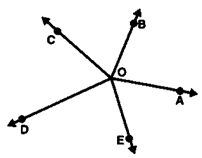Solution:
It is given that rays the endpoint O is shared by OA, OB, OC, OD, and OE.
By the construction we build a straight line AS by drawing an opposite ray OS to ray OA.From the construction we know that AS is line.
So, ∠AOB and ∠BOS are linear pair angles
∠BOS = ∠BOC + ∠COS
∠AOB + ∠BOS = 180°
∠AOB + ∠BOC + ∠COS = 180° __________(1)
So, ∠AOE and ∠EOS are linear pair angles
∠EOS = ∠DOE + ∠DOS
∠AOE + ∠EOS =180°
Or, ∠AOE + ∠DOE + ∠DOS = 180° __________(2)
∠AOB + ∠BOC + ∠COF + ∠AOE + ∠DOE + ∠DOS = 180° + 180°
∠AOB + ∠BOC + ∠COD + ∠DOE + ∠EOA = 360°
Hence, it is proved that ∠AOB + ∠BOC + ∠COD + ∠DOE + ∠EOA = 360°.

Question 5: In figure, ∠AOC and ∠BOC form a linear pair. If a – 2b = 30°, find a and b?Solution:
It is given that,
a – 2b = 30° __________(1)
∠AOC and ∠BOC form a linear pair
a + b = 180° __________(2)
By subtracting equation (1) from (2),
a + b – a + 2b = 180 – 30
3b = 150
b = 150/3
b = 50°
Put the value of b in equation.
a – 2b = 30°
a – 2(50) = 30
a – 100 = 30
a = 30 + 100
a = 130°
Hence, the values of a and b are 130° and 50°.

Question 6: How many pairs of adjacent angles are formed when two lines intersect at a point?
Solution: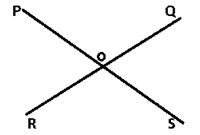The 4 pair of adjacent angles are:
∠POS, ∠SOQ
∠SOQ, ∠QOR
∠ROP, ∠POS
∠QOS, ∠ROP

Question 7:  How many pairs of adjacent angles, in all, can you name in figure given?Solution:
Adjacent angles, from the figure, are:
∠EOC and ∠DOC
∠EOD and ∠DOB
∠DOC and ∠COB
∠EOD and ∠DOA
∠DOC and ∠COA
∠BOC and ∠BOA
∠BOA and ∠BOD
∠BOA and ∠BOE
∠EOC and ∠COA
∠EOC and ∠COB
Thus, the above 10 are adjacent angles.

Question 8: In figure, determine the value of x.Solution:
360° is equal to the number of all angles at a point O.
3x+3x+150+x=360°
7x=360°–150°
7x = 210°
x=210/7
x=30°
Hence, the value of x is 30°.

Question 9:  In figure, AOC is a line, find x.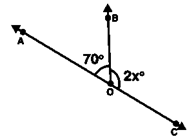Solution:
It is given that,
∠AOB + ∠BOC = 180° (linear pairs)
70 + 2x = 180
2x = 180 – 70
2x = 110
x =  110/2
x = 55
Hence,the value of x is 55°.

Question 10: In figure, POS is a line, find x.Solution:
∠POQ + ∠QOS = 180° (linear pairs)
∠POQ + ∠QOR + ∠SOR = 180°
600 + 4x + 400 = 180°
4x = 180° - 100°
4x = 80°
x = 20°
Thus, the value of x is 20°.

Question 11:  In the below figure, ACB is a line such that ∠DCA = 5x and ∠DCB = 4x. Find the value of x?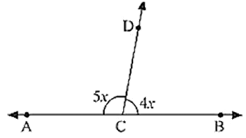Solution:
It is given that,
∠DCA = 5x and ∠DCB = 4x
As, AB is a line
∠ACD + ∠BCD = 180° (linear pairs)
5x+4x=180
9x=180
x=20
Hence, the value of x is 20°

Question 12: In the given figure, Given ∠POR = 3x and ∠QOR = 2x + 10, Find the value of x for which POQ will be a line?Solution:
POR is a Straight line.
It is given that,
∠POR = 3x
∠QOR = 2x + 10
∠POR + ∠QOR = 180° (Linear Pair)
3x + 2x + 10 = 180
5x + 10 = 180
5x = 180 – 10
5x = 170
x = 170/5
x = 34
Hence the value of x is 34°

Question 13: In Fig: a is greater than b by one third of a right angle. Find the value of a and b?Solution:
AB is a straight line
So, a + b = 180
a = 180 – b _________(1)
It is given that,
a is greater than b by one third of a right angle
a = b + 90/3
a = b + 30_________(2)
Put both the equations Equal.
180 – b = b + 30
180 – 30 = 2b
b = 150/2
b = 75
Put the value of b in equation (1)
a = 180 – b
a = 180 – 75
a = 105
Thus, the values of a and b are 105° and 75°.

Question 14: What value of y would make AOB a line in the below figure, If ∠AOB = 4y and ∠BOC = (6y + 30) ?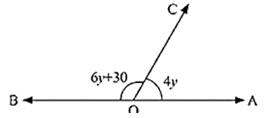Solution:
AB is a straight line,
∠AOC + ∠BOC = 180° (Linear pairs)
4y + 6y + 30 = 180
10y + 30 = 180
10y = 180 - 30
10y = 150
y = 150/10
y = 15
Thus, the value of y is 15°

Question 15: If the figure below forms a linear pair, forms a linear pair ∠EOB = ∠FOC = 90° and ∠DOC = ∠FOG = ∠AOB = 30°. Find the measure of ∠FOE, ∠COB and ∠DOE
Name all the right angles
Name three pairs of adjacent complementary angles
Name three pairs of adjacent supplementary angles
Name three pairs of adjacent anglesSolution:
(i) It is given that,
Let us assumed that,
∠FOE = x, ∠DOE = y and ∠BOC = z
AG is a straight line,
∠AOF + ∠FOG = 180 (Linear pair)
∠AOF + 30 = 180
∠AOF = 180 - 30
∠AOF = 150
We know that,
∠AOF = ∠AOB + ∠BOC + ∠COD + ∠DOE + ∠EOF
∠AOB + ∠BOC + ∠COD + ∠DOE + ∠EOF = 150
30 + z + 30 + y + x = 150
x + y + z =150 - 30 - 30
x + y + z = 90 ________(1)
It is given that,
∠FOC = 90°
We know that
∠FOC = ∠FOE + ∠EOD + ∠DOC
∠FOE + ∠EOD + ∠DOC = 90°
x + y + 30 = 90
x + y = 90 - 30
x + y = 60 ________ (2)
Put the value of x + y in equation (1)
x + y + z = 90
60 + z = 90
z = 90 - 60
z = 30
It is given that ∠BOE = 90
∠BOC + ∠COD + ∠DOE = 90°
30 + 30 + ∠DOE = 90
∠DOE = 90 - 60 = 30
∠DOE = 30
x = 30
Put the value of x in equation (2)
x + y = 60
y = 60 - x
y = 60 - 30
y = 30
Thus, ∠FOE = 30°, ∠COB = 30° and ∠DOE = 30°

(ii) ∠DOG, ∠COF, ∠BOF, ∠AOD are the Right angles.

(iii) ∠AOB, ∠BOD
∠AOC, ∠COD
∠BOC, ∠COE
Above are the adjacent complementary angles.

(iv) ∠AOB, ∠BOG
∠AOC, ∠COG
∠AOD, ∠DOG
Above are the adjacent supplementary angles.

(v) ∠BOC, ∠COD
∠COD, ∠DOE
∠DOE, ∠EOF

Question 16:  In below fig. OP, OQ, OR and OS are four rays. Prove that: ∠POQ + ∠QOR + ∠SOR + ∠POS = 360°.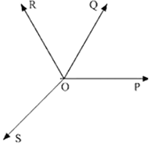Solution:∠XOR+∠ROP=180° (Linear Pair)
∠ROP=∠ROQ+∠QOP
∠XOR+∠ROQ+∠QOP=180° __________(1)
∠XOS+∠SOP=180° (Linear Pair) __________(2)
∠XOR+∠ROQ+∠QOP+∠XOS+∠SOP=180°+180°
∠XOR+∠ROQ+∠QOP+∠XOS+∠SOP=360°
We know that,
∠SOR=∠XOR+∠XOS
∠SOR+∠ROQ+∠QOP+∠SOP=360°
Hence Proved.

Question: 17: In below fig, ray OS stand on a line POQ. Ray OR and ray OT are angle bisectors of ∠POS and ∠SOQ respectively. If ∠POS = x, find ∠ROT?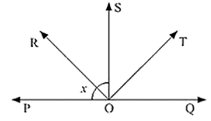Solution: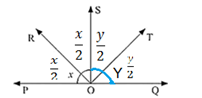∠POS+∠SOQ=180° (Linear Pair)
∠POR+∠ROS+∠SOT+∠TOQ=180°
x/2+x/2+y/2+y/2=180°
(x+x+y+y)/2=180°
2x+2y=2×180°
2x+2y=360°
2(x+y)=360°
x+y=(360°)/2
x+y=180°
We know that,
∠ROT = x/2+y/2
∠ROT = (x + y )/2
∠ROT = (180° )/2
∠ROT = 90°
Hence, the value of ∠ROT is 90°.

Question 18: In the below fig, lines PQ and RS intersect each other at point O. If ∠POR: ∠ROQ = 5: 7. Find all the angles.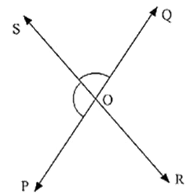Solution:
It is given that,
∠POR : ∠ROQ = 5:7
∠POR + ∠ROP = 180° (linear pair)
∠POR = 180 × 5/12
∠POR = 75°
∠ROQ = 180 × 5/12
∠ROQ = 105°
So,
∠POS = ∠ROQ = 105° (Vertically opposite angles)
∠SOQ = ∠POR = 75° (Vertically opposite angles)
Hence, the angles will be ∠POS = ∠ROQ = 105° and ∠SOQ = ∠POR = 75°.

Question 19: In the below fig. POQ is a line. Ray OR is perpendicular to line PQ. OS is another ray lying between rays OP and OR. Prove that ∠ROS = 1/2(∠QOS − ∠POS).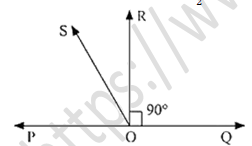Solution:
It is given that,
OR perpendicular
∠POS+∠SOR=90°
∠POS+∠SOQ=180° (Linear Pair)
Multiplying by 2 we get,
2(∠POS+∠SOR)=2×90°
2∠POS+2∠SOR=180°
∠POS+∠SOQ=2∠POS+2∠SOR
∠SOQ-2∠SOR=2∠POS-∠POS
∠SOQ-∠POS=2∠ROS
(∠SOQ-∠POS)/2=∠ROS
1/2(∠SOQ-∠POS)=∠ROS
∠ROS=1/2(∠SOQ-∠POS)
Hence Proved.

Exercise 8.3

Question 1: In figure, lines l1, and l2 intersect at O, forming angles as shown in the figure. If x = 45. Find the values of y, z and u.Solution:
It is given that x = 45°
z = x = 45° (vertically opposite angles) ___________(1)
From Line l1 we get,
z + u = 180° (linear pair) _______(2)
Put the value of z in equation (2).
z + u = 180°
45° + u = 180°
u = 180° – 45°
u = 135°
u = y = 135° (vertically opposite angles)
Hence, the angles are y = 135°, u = 135° and z = 45°.

Question 2: In figure, three coplanar lines intersect at a point O, forming angles as shown in the figure. Find the values of x, y, z and u.Solution:
It is given that,
∠BOD = 90° and ∠DOF = 50°
So,
∠BOD = z = 90° (Vertically opposite angles)
∠DOF = y = 50° (Vertically opposite angles)
AB is a straight line,
x + y + z = 180 (Linear pair)
x + y + z = 180
x + 50 + 90 = 180
x = 180 – 140
x = 40
Thus, the values of x, y, z and u are 40°, 50°, 90° and 40°.

Question 3:  In figure, find the values of x, y and z.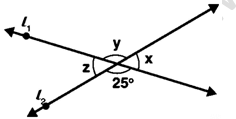Solution:
y = 25° (Vertically opposite angles)
l1 is a straight line,
x + y = 180° (Linear pair of angles)
x = 180° – 25°
x = 155°
l2 is a straight line,
z + 25° = 180° (Linear pair of angles)
z = 180° – 25°
z = 155°
Hence, the value of x, y and z are 155°, 25° and 155°

Question 4: In figure, find the value of x.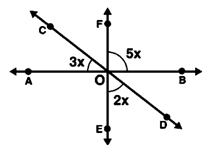Solution:
It is given that,
∠BOF = 5x
∠AOE = 3x
∠EOD = 2x
∠AOE = ∠BOF = 5x (Vertically opposite angles)
CD is a straight line,
∠COA + ∠AOE + ∠FOC = 180° [Linear pair]
3x + 5x + 2x = 180
10x = 180
x = 180/10
x = 18°
Hence, the value of x is 18°

Question 5: Prove that bisectors of a pair of vertically opposite angles are in the same straight line.
Solution: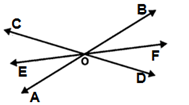We know that,
∠BOD = ∠COA (Vertically angles)
∠FOB + ∠BOE = 180° (Linear Pair)
∠FOB + ∠BOC + ∠COE =180° __________(1)
∠EOC + ∠COF = 180° (Linear Pair)
∠ EOC + ∠COB + ∠BOF =180° __________(2)
EOF is a Straight line.
Hence Proved

Question 6: If two straight lines intersect each other, prove that the ray opposite to the bisector of one of the angles thus formed bisects the vertically opposite angle.
Solution: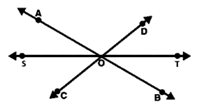It is given that,
Straight lines AB and CD intersect each other at O.
Here, OS is the bisector of ∠AOC.
Straight lines AB, CD and ST are intersect each other at O.
∠AOS = ∠BOT (Vertically opposite angles)
∠COS = ∠DOT (Vertically opposite angles)
OS is the bisector of ∠AOC
So, ∠AOS = ∠COS
Thus, ∠BOT = ∠ DOT
Therefore, OT is the bisector of ∠BOD.

Question 7:  If one of the angles formed by intersecting lines is an angle then show that the each of the angles is a right angle.
Solution: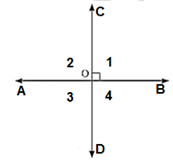It is given that,
∠1=90°
∠AOB=90°
∠1+∠2=180° (Linear Pair)
90°+∠2=180°
∠2=180°-90°
∠2=90°
∠COD=180°
∠2+∠3=180° (Linear Pair)
90°+∠3=180°
∠3=180°-90°
∠3=90°
∠1+∠4=180° (Linear Pair)
90°+∠4=180°
∠4=180°-90°
∠4=90°
∠1+∠2+∠3+∠4=90°
Hence, ∠AOC=∠BOC=∠BOD=∠AOD=90°

Question: 8: In the below fig. rays AB and CD intersect at O.
(i)  Determine y when x = 60
(ii)  Determine x when y = 40Solution:
(i) Determine y when x=60°
∠AOC + ∠COB = 180°(Linear Pair)
2x + y = 180°
2(60) + y = 180°
120° + y = 180°
y=180°-120°
y=60°

(ii)  Determine x when y=40°
∠AOC + ∠COB = 180°(Linear Pair)
2x + y = 180°
2x + 40 = 180°
2x=180°-40°
2x=140°
x= (140°)/2
x=70°

Question 9:  In the below fig. lines AB. CD and EF intersect at O. Find the measures of ∠AOC, ∠COF, ∠DOE and ∠BOF.
Solution: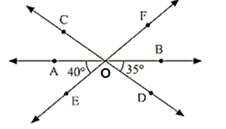Solution:
It is given that,
∠AOE + ∠EOB = 180°(Linear Pair)
∠AOE + ∠DOE + ∠DOB = 180°
∠AOE + ∠DOE + 35° = 180°
40° + ∠DOE + 35° = 180°
∠DOE + 75° = 180°
∠DOE = 180°-75°
∠EOD = 105°
∠AOE = ∠FOB = 40°(Vertically Opposite angle)
∠BOD = ∠AOC = 35°(Vertically Opposite angle)
∠DOE = ∠COF = 105°(Vertically Opposite angle)
Hence, the value of ∠AOC, ∠COF, ∠DOE and ∠BOF are 35°, 105°, 105°, and 40° respectively.

Question 10: AB, CD and EF are three concurrent lines passing through the point O such that OF bisects BOD.  If BOF = 35. Find BOC and AOD.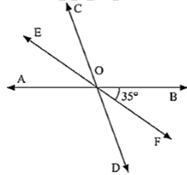Solution:
It is given that,
OF bisects BOD
∠BOF = 35°
∠BOF = ∠FOD (OF is bisects BOD)
∠BOF = ∠FOD = 35°
∠BOF + ∠FOD = ∠BOD
35° + 35° = ∠BOD
70° = ∠BOD
∠BOD = ∠AOC = 70° (Vertically opposite angles]
∠BOC + ∠AOC = 180° (Linear Pair)
∠BOC + 70° = 180°
∠BOC = 180° - 70°
∠BOC = 110°
∠AOD = ∠BOC = 110° (Vertically opposite angles)

Question 11:  In below figure, lines AB and CD intersect at O. If ∠AOC + ∠BOE = 70° and ∠BOD = 40°, find ∠BOE and reflex ∠COE?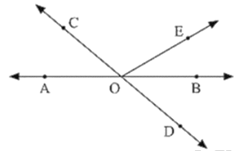Solution:
It is given that,
∠AOC + ∠BOE = 70° and ∠BOD = 40°
∠BOD = ∠AOC (vertically opposite angles)
∠BOD = 40°
∠BOD = ∠AOC = 40°
∠AOC + ∠BOE = 70°,
40° + ∠BOF = 70°
∠BOF = 70° - 40°
∠BOE = 30°
∠AOC + ∠COE + ∠BOE = 180° (Lines pair)
40° + ∠COE + 30° = 180°
∠COE = 180° - 30° - 40°
∠COE = 110°
Hence, Reflex ∠COE = 360° - 110° = 250°.

Question 12:  Which of the following statements are true (T) and which are false (F)?
(i) Angles forming a linear pair are supplementary.
(ii) If two adjacent angles are equal and then each angle measures 90
(iii) Angles forming a linear pair can both acute angles.
(iv) If angles forming a linear pair are equal, then each of the angles have a measure of 90
Solution:
(i) True
(ii) False
(iii) False
(iv) true

Question 13:  Fill in blanks so as to make the following statements true:
(i) If one angle of a linear pair is acute then its other angle will be______
(ii) A ray stands on a line, then the sum of the two adjacent angles so formed is ______
(iii) If the sum of two adjacent angles is 180, then the ______ arms of the two angles are opposite rays.
Solution:
(i) If one angle of a linear pair is acute then its other angle will be Obtuse angle.
(ii) A ray stands on a line, then the sum of the two adjacent angles so formed is 180°
(iii) If the sum of two adjacent angles is 180, then the Uncommon arms of the two angles are opposite rays.

Exercise 8.4

Question 1:  In figure, AB, CD and ∠1 and ∠2 are in the ratio 3 : 2. Determine all angles from 1 to 8.Solution:
It is given that,
∠1 and ∠2 are in the ratio 3 : 2
It is assumed that,
∠1=3x
∠2=2x
∠1+∠2=180° (Linear pair)
3x+2x=180°
5x=180°
x=180/5
x=36°
Thus,
∠1=3x
∠1=3 × 36°
∠1=108°
and
∠2=2x
∠2=2×36°
∠2=72°
∠1 = ∠3 = 108° (vertically opposite angles)
∠2 = ∠4 = 72° (vertically opposite angles)
We also know, if a transversal intersects any parallel lines, then the corresponding angles are equal
∠1 = ∠5 = 108° (corresponding angles)
∠2 = ∠6 = 72° (corresponding angles)
So,
∠5 = ∠7 = 108° (vertically opposite angles)
∠6 = ∠8 = 72° (vertically opposite angles)
Hence, the value of angles ∠1 = 108°, ∠2 = 72°, ∠3 = 108°, ∠4 = 72°, ∠5 = 108°, ∠6 = 72°, ∠7 = 108° and ∠8 = 72°.

Question 2:  In figure, I, m and n are parallel lines intersected by transversal p at X, Y and Z respectively. Find ∠1, ∠2 and ∠3.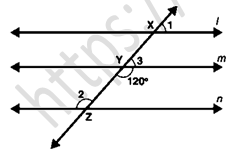Solution:
As per figure we find that,
∠Y = 120° (Vertical opposite angles)
∠3 + ∠Y = 180° (Linear pair angles)
∠3 = 180° – 120°
∠3 = 60°
As Shown in figure,
Line l is parallel to line m,
∠1 = ∠3 (Corresponding angles)
∠1 = 60°
Line m is parallel to line n,
∠2 = ∠Y (Alternate interior angles)
∠2 = 120°
∠2 = ∠Z (Vertical opposite angles)
∠Z = 120°
Hence, the value of angle ∠1 = 60°, ∠2 = 120° and ∠3 = 60°.

Question 3:  In figure, AB || CD || EF and GH || KL. Find ∠HKL.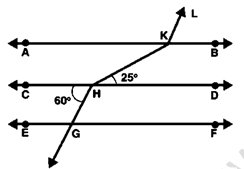Solution:
Extend LK to meet line GF at point R.It is given that,
∠KHY = 25°
∠KHY = ∠XKH = 25° (Alternate interior angles)
∠KHC = ∠CHG = 180° (Linear Pair)
∠XHC + 60° = 180°
∠XHC = 180°- 60°
∠XHC = 120°
∠CHG = ∠AXH = 60° (Corresponding angles)
∠AXH = ∠XKY = 60° (Corresponding angles)
∠XKY = ∠XKL = 180° (Linear Pair)
60° + ∠XKL = 180°
∠XKL = 180°- 60°
∠XKL = 120°
∠HKL = ∠LKX + ∠XKH
∠HKL = 120° + 25°
∠HKL = 145°
Hence, the value of ∠HKL is 145°.

Question 4: In figure, show that AB || EF.Solution:
∠BAC = ∠ACD (Alternate interior angle)
∠BAC = ∠ACD = 57°
∠ACD = ∠ACE + ∠ECD
∠ACD = 22° + 35°
∠ACD = 57°
So, BA||CD___________(1)
∠FEC + ∠ECD = 180° (Interior angles on the same side)
145° + 35° = 180°
So, CD||EF___________(1)
From equation 1 and 2 we get,
AB||CD||EF
Hence we can say that, AB||EF.
Hence Proved

Question 5 :  In figure, if AB || CD and CD || EF, find ∠ACE.Solution:
It is given that, AB|| EF
AB||EF||CD
∠BAC = ∠ACD (Alternate interior angles)
∠BAC = ∠ACD = 70°
∠FEC = ∠ECD = 180°
130° + ∠ECD = 180°
∠ECD = 180° - 130°
∠ECD = 50°
∠ACD = ∠ACE + ∠ECD
70° = ∠ACE + 50°
∠ACE = 70° + 50°
∠ACE = 20°
Hence, the ∠ACE is 20°.

Question 6: In figure, PQ || AB and PR || BC. If ∠QPR = 102°, determine ∠ABC. Give reasons.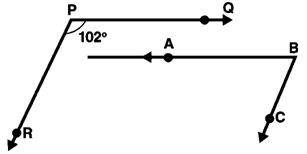Solution: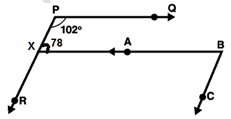It is given that,
∠QPR = 102°
∠QPX + ∠PXA = 180° (sum of interior angles on the same side)
102° + ∠PXA = 180°
∠PXA = 180°- 102°
∠PXA = 78°
Also given that, PR||BC
∠PXA = ∠ABC = 78° (Alternate Interior angle)
Hence, the value of ∠ABC is 78°.

Question 7: In figure, state which lines are parallel and why?Solution:
∠EDC = ∠D (Vertically Opposite angles)
∠EDC = ∠D = 100°
∠ACD = ∠D (Corresponding angles)
∠ACD = ∠D = 100°
Corresponding angles are only made by parallel lines.
Hence, we can say that DE || AC.

Question 8:  In figure, if l||m, n || p and ∠1 = 85°, find ∠2.Solution:
It is given that,
∠1 = 85°
∠1 = ∠3 = 85° (Corresponding angles)
Again, co-interior angles are supplementary, so
∠3 + ∠2 = 180° (interior angles of the same side of a transversal)
85° + ∠2 =180°
∠2 = 180° – 85°
∠2 = 95°
Hence, the value of ∠2 is 95°.

Question 9: If two straight lines are perpendicular to the same line, prove that they are parallel to each other.
Solution:l and m are straight lines.
n ⊥ l
And n ⊥ m
∠1 = ∠2 (Corresponding angles)
∠1 = ∠2 = 90°
l || m
Hence Prove

Question 10: Prove that if the two arms of an angle are perpendicular to the two arms of another angle, then the angles are either equal or supplementary.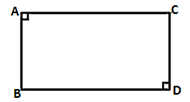Solution:
It is assumed that
The angles be ∠ACB and ∠ABD
AC perpendicular to AB,
CD is perpendicular to BD.
∠A+ ∠C+ ∠D+ ∠B = 360° (Sum of angles in a quadrilateral is 360°)
180° + ∠C + ∠B = 360°
∠C + ∠B = 360° –180°
Therefore,
∠ACD + ∠ABD = 180°
∠ABD = ∠ACD = 90° (Angles are equal and supplementary)
Hence Proved,

Question: 11: In the below fig, lines AB and CD are parallel and P is any point as shown in the figure. Show that ∠ABP + ∠CDP = ∠DPB.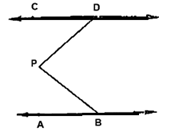Solution:It is given that AB||CD
Draw a line MN parallel to AB or CD
AB||MN||CD
CD||MN
∠4 = ∠1 (Alternate interior angles)
MN||AB
∠2 = ∠3 (Alternate interior angles)
∠DPB = ∠1 + ∠2
∠DPB = ∠4 + ∠3
∠DPB = ∠CDP + ∠ABP
Hence Prove

Question: 12: In the below fig, AB ∥ CD and P is any point shown in the figure. Prove that: ∠ABP + ∠BPD + ∠CDP = 360°Solution: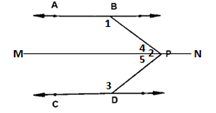We can draw a line MN parallel to AB or CD through P.
AB|| MN
∠1 + ∠4 = 180°____________(1) (sum of two sides of same side of transversal)
MN||CD
∠5 + ∠3 = 180°____________(2) (sum of two sides of same side of transversal)
From the fig. we get,
∠2 = ∠4 + ∠5
By adding equation (1) and (2) we get,
∠1 + ∠4 + ∠5 + ∠3 = 180° + 180°
∠1 + ∠2 + ∠3 = 360°
∠ABP + ∠BPD + ∠CDP = 360°
Hence Prove

Question: 13:  Two unequal angles of a parallelogram are in the ratio 2: 3. Find all its angles in degrees.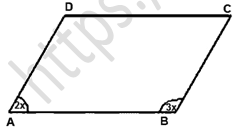Solution:
Let us assumed that,
∠A = 2x
∠B = 3x
∠A + ∠B = 180° __________(1) (Co-interior angles are supplementary)
Put the value of ∠A and ∠B in equation (1)
2x + 3x = 180°
5x = 180°
x = (180°)/5
x = 36°
A = 2 × 36 = 72°
B = 3 × 36 = 108°
A = C = 72° (Opposite side angles and sides of a parallelogram are equal)
B = D = 108° (Opposite side angles and sides of a parallelogram are equal)
Hence Prove.

Question: 14:  If each of the two lines is perpendicular to the same line, what kind of lines are they to each other?Solution:
Let us assumed that,
AB ⊥ MN
∠ABD = 90°_______(i)
CO ⊥ MN
∠CON = 90°_______ (ii)
∠AYX = 90°
∠CDN = 90°
∠1 = ∠2 (Corresponding angle)
AB || CD,
Hence Prove.

Question: 15:  In the below fig, ∠1 = 60° and ∠2 = 2/3rd of a right angle. Prove that l ∥ m.Solution:
It is given that,
∠1 = 60° and ∠2 = 2/3 rd of a right angle.
According to figure m is parallel to l.
∠2 = (2/3) × 90°
∠2 = 60°
∠1 = ∠2 (Corresponding angles)
∠1 = 60°
Hence, Parallel to m as pair of corresponding angles are equal.

Question: 16: In the below fig, if l ∥ m ∥ n and ∠1 = 60°. Find ∠2.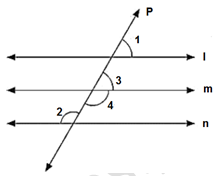Solution:
It is given that l || m ||n
∠1 = 60°
∠1 = ∠3 = 60° (Corresponding angles)
∠3 + ∠4 = 180° (linear pair)
60° + ∠4 = 180°
∠4 = 180° - 60°
∠4 = 120°
m ∥ n and P is the transversal
∠4 = ∠2
∠2 = 120° (Alternative interior angle)
Hence, the value of ∠2 is 120°.

Question: 17:  Prove that the straight lines perpendicular to the same straight line are parallel to one another.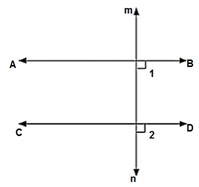Solution:
It is assumed that,
AB and CD perpendicular to MN
∠ABD = 90° (AB is perpendicular to MN)_________(i)
∠CON = 90° (CD is perpendicular to MN)________(ii)
From the equation we get,
∠ABD = ∠CDN = 90°
Hence, Corresponding angles are equal AB||CD.

Question: 18: The opposite sides of a quadrilateral are parallel. If one angle of the quadrilateral is 60°. Find the other angles.Solution:
It is given that,
AB || CD
Co-interior angles are supplementary
∠A + ∠D = 180° (Co-interior angles are supplementary)
60° + ∠D = 180°
∠D = 180° - 60°
∠D = 120°
Co-interior angles are supplementary
∠A + ∠B = 180° (Co-interior angles are supplementary)
60 + ∠B = 180°
∠B = 180° - 60°
∠B = 120°
Hence, the value of ∠A = 60° and ∠B = ∠D = 120°

Question: 19:  Two lines AB and CD intersect at O. If ∠AOC + ∠COB + ∠BOD = 270°, find the measures of ∠AOC, ∠COB, ∠BOD, ∠DOA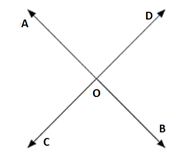Solution:
It is given that, ∠AOC + ∠COB + ∠BOD = 270°
∠AOC + ∠COB + ∠BOD + ∠AOD = 360° (Complete angle)
270° + ∠AOD = 360°
∠AOD = 360° - 270°
∠AOD = 90°
∠AOD + ∠BOD = 180° (Linear pair)
90° + ∠BOD = 180°
∠BOD = 180° - 90°
∠BOD = 90°
∠AOD = ∠BOC = 90 ° (Vertically opposite angles)
∠BOD = ∠AOC = 90° (Vertically opposite angles)
Hence, the value of ∠AOD, ∠BOC, ∠BOD and ∠AOC is 90°.

Question: 20: In the below figure, p is a transversal to lines m and n, ∠2 = 120° and ∠5 = 60°. Prove that m|| n.Solution:
It is given that, ∠2 = 120° and ∠5 = 60°
∠2 + ∠1 = 180° (Linear pair)
120° + ∠1 = 180°
∠1 = 180° − 120°
∠1 = 60°
∠1 = ∠5 = 60°
Hence, m || n (corresponding angles are equal)

Question: 21:  In the below fig. transversal t intersects two lines m and n, ∠4 = 110° and ∠7 = 65° is m ∥ n?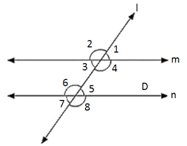Solution:
It is given that, ∠4 = 110° and ∠7 = 65°
∠7 = ∠5 = 65° (Vertically opposite angle)
∠4 + ∠5 = 180° (co interior angles is supplementary angle)
110° + 65° = 175°
Hence, here m is not parallel to n as the pair of co interior angles is not supplementary.

Question: 22:  Which pair of lines in the below fig. is parallel? Give reasons.Solution:
∠A + ∠B = 180°
115° + 65° = 180°
∠B + ∠C = 180°
65° + 115° = 180°
The sum of interior angles are supplementary Hence, AB||CD

Question: 23:  If I, m, n are three lines such that I || m and n perpendicular to l, prove that n perpendicular to m.Solution: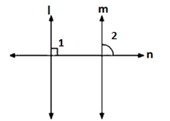It is given that l || m, n perpendicular to I
l || m and n intersecting line
∠1 = ∠2 (Corresponding angles)
∠2 = 90° (as per fig.)
Thus, we can say that n is perpendicular to m.

Question: 24:  In the below fig, arms BA and BC of ∠ABC are respectively parallel to arms ED and EF of ∠DEF. Prove that ∠ABC = ∠DEF.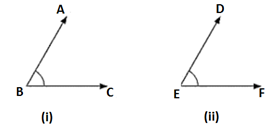Solution:
It is given that AB ∥ DE and BC ∥ EF
By the construction we get BC to x such that it intersects DE at M.
AB ∥ DE and BX is the transversal
∠ABC = ∠DMX (Corresponding angle)________(i)
BX ∥ EF and DE is the transversal
∠DMX = ∠DEF (Corresponding angles) ________ (ii)
From equation (i) and equation (ii) we get,
∠ABC = ∠DEF
Hence Proved.

Question: 25:  In the below fig, arms BA and BC of ABC are respectively parallel to arms ED and EF of DEF Prove that
∠ABC + ∠DEP = 180°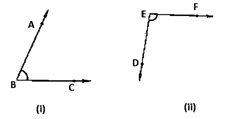Solution:
It is given that,
AB ∥ DE, BC ∥ EF
By the construction we get BC to intersect DE at M
AB || EM and BL is the transversal
∠ABC = ∠EML (Corresponding angle)__________(i)
EF || ML and EM is the transversal
∠DEF + ∠EML = 180° (co-interior angles are supplementary)________(ii)
From equation (1) and (2) we get,
∠DEF + ∠ABC = 180°
Hence Proved.

Question: 26:  With of the following statements are true (T) and which are false (F)? Give reasons.
(i) If two lines are intersected by a transversal, then corresponding angles are equal.
(ii) If two parallel lines are intersected by a transversal, then alternate interior angles are equal.
(ii) Two lines perpendicular to the same line are perpendicular to each other.
(iv) Two lines parallel to the same line are parallel to each other.
(v) If two parallel lines are intersected by a transversal, then the interior angles on the same side of the transversal are equal.
Solution:
(i) False
(ii)True
(iii) False
(iv) True
(v) False

Question: 27:  Fill in the blanks in each of the following to make the statement true:
(i) If two parallel lines are intersected by a transversal, then each pair of corresponding angles are ____________
(ii) If two parallel lines are intersected by a transversal, then interior angles on the same side of the transversal are _____________
(iii) Two lines perpendicular to the same line are _______ to each other
(iv) Two lines parallel to the same line are __________ to each other.
(v) If a transversal intersects a pair of lines in such a way that a pair of alternate angles we equal. then the lines are ___________
(vi) If a transversal intersects a pair of lines in such a way that the sum of interior angles on the seine side of transversal is 180'. then the lines are _____________
Solution:
(i) Equal
(ii) Parallel
(iii) Supplementary
(iv) Parallel
(v) Parallel
(vi) Parallel

Exercise VSAQs.......................

Question 1: Define complementary angles.
Solution:
Two angles are said to be complementary, if the sum of their measures is 90°.

Question 2: Define supplementary angles.
Solution:
Two angles are said to be complementary, if the sum of their measures is 180°.

Solution:
Two angles are Adjacent when they have a common side and a common vertex.

Question 4: The complement of an acute angle is _____.
Solution:
The complement of an acute angle is an acute angle.

Question 5: The supplement of an acute angle is _____.
Solution:
The supplement of an acute angle is an obtuse angle.

Question 6:  The supplement of a right angle is _____.
Solution:
The supplement of a right angle is a right angle.